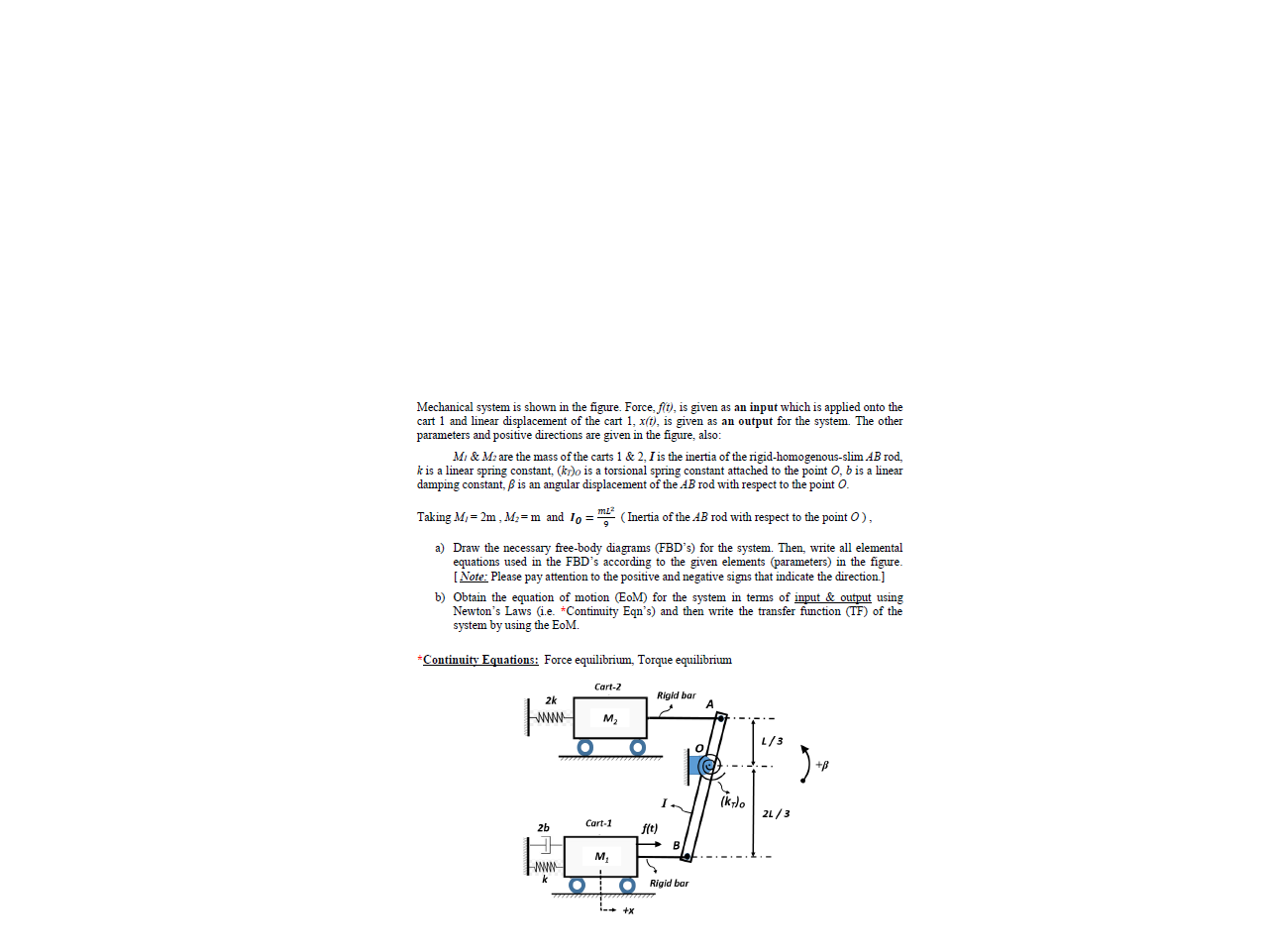# 2) Draw the necessary free body diagrams (FBD's) for the system. Then, write all elemental equations used in the FED's according to the given elements (parameters) in the figure. INete, Please pay attention to the positive and negative signs that indicate the direction] b) Obtain the equation of motion (EoM) for the system in terms of ingut & output using Newton's Laws (Le *Contimity Eqn's) and then write the transfer flunction (TF) of the system by using the EoM. Continuiry Equations: Force equilibrium, Torque equilibrium

Question

i need very fast answer because i have not more timehelp_outlineImage Transcriptionclose2) Draw the necessary free body diagrams (FBD's) for the system. Then, write all elemental equations used in the FED's according to the given elements (parameters) in the figure. INete, Please pay attention to the positive and negative signs that indicate the direction] b) Obtain the equation of motion (EoM) for the system in terms of ingut & output using Newton's Laws (Le *Contimity Eqn's) and then write the transfer flunction (TF) of the system by using the EoM. Continuiry Equations: Force equilibrium, Torque equilibrium fullscreen

### Want to see this answer and more?

Experts are waiting 24/7 to provide step-by-step solutions in as fast as 30 minutes!*

*Response times may vary by subject and question complexity. Median response time is 34 minutes for paid subscribers and may be longer for promotional offers.
Tagged in
Engineering
Mechanical Engineering

### Mechanical Vibrations